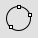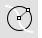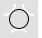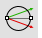# Circle toolbar

#####Circle

Draw a circle from center and radius, diameter, points on the circumference, and circumference length.

#####Circle, Diameter

Draws a circle from points on diameter.

#####Circle, 3Point

Draws a circle from three points on circumference.

#####Circle, AroundCurve

Draws a circle around a curve.

#####Circle, Tangent, Tangent, Radius

Draws a circle tangent to two curves and a radius.

#####Circle, Tangent

Draws a circle tangent to 3 curves.

#####Circle, Vertical to CPlane: center, radius

Draws a circle from a center and radius vertical to CPlane.

#####Circle, Vertical to CPlane: diameter

Draws a circle from points on diameter vertical to CPlane.

#####Circle, Deformable

Draws a deformable NURBS circle.

#####Circle, Fit Points

Fits a circle through points.

Rhinoceros 7 © 2010-2022 Robert McNeel & Associates. 04-Jul-2022